## Example Questions

### Example Question #114 : Coordinate Geometry

What is the slope of the line whose equation is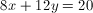?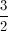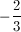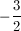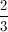Explanation:

Solve for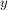so that the equation resembles the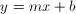form. This equation becomes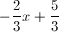. In this form, theis the slope, which is.

### Example Question #1 : How To Find The Equation Of A Curve

Which of the following equations has a-intercept of?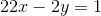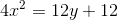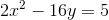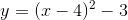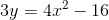Explanation:

To find the-intercept, you need to find the value of the equation where.  The easiest way to do this is to substitute infor your value ofand see where you getfor.  If you do this for each of your equations proposed as potential answers, you find thatis the answer.

Substitute infor: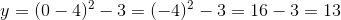### Example Question #111 : Coordinate Geometry

Ifis a line that has a-intercept ofand an-intercept of, which of the following is the equation of a line that is perpendicular to?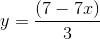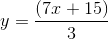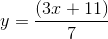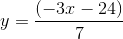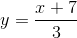Explanation:

Ifhas a-intercept of, then it must pass through the point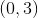.

If its-intercept is, then it must through the point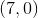.

The slope of this line is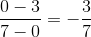.

Therefore, any line perpendicular to this line must have a slope equal to the negative reciprocal, which is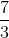. Onlyhas a slope of.

### All GRE Math Resources